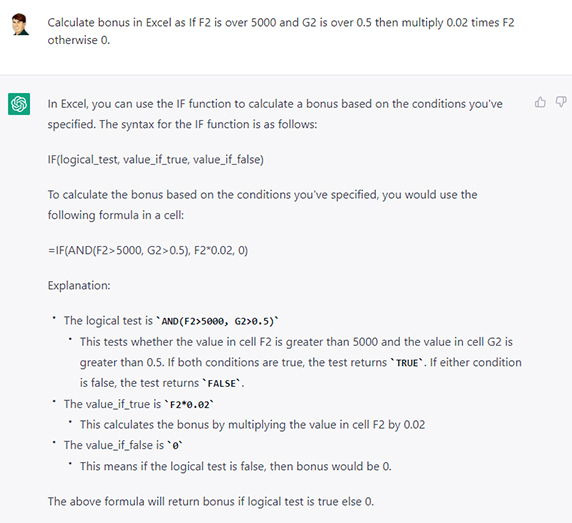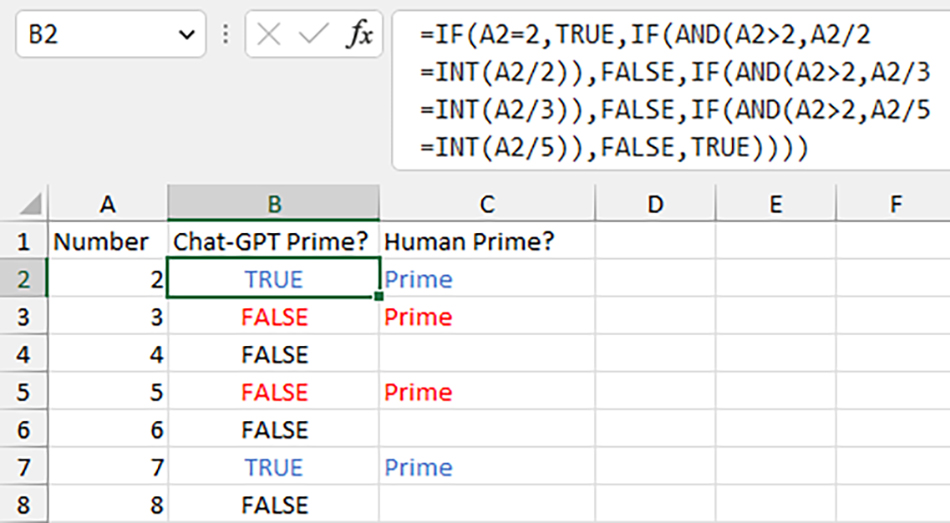ChatGPT is able to produce natural, human-like text, but questions arise as to its accuracy and concerns over how the technology could be used. The concerns over accuracy extend even into use for Excel.

After several people asked me about using ChatGPT to write Excel formulas, VBA code, or Power Query M code, I conducted several tests in January 2023 and found that it isn’t quite yet ready.

The first result I received was amazing. I have an example in my Power Excel seminar where I show how to handle an IF function with two conditions. I asked ChatGPT to “Calculate bonus in Excel as If F2 is over 5000 and G2 is over 0.5 then multiply 0.02 times F2 otherwise 0.” In a few seconds, it produced the correct answer of =IF(AND(F2>5000,G2>0.5),F2*0.02,0). That’s better than the more common =IF(F2>5000,IF(G2>0.5,F2*0.02,0)), and I was impressed that ChatGPT offered the better formula. ChatGPT even gave a short tutorial on how the IF function works (see Figure 1).Then I asked a more difficult question: “Excel formula to test if the value in A2 is a prime number.” In just a few seconds, ChatGPT confidently presented this formula with a caution that it would only work on small numbers:

=IF(A2=2,TRUE,IF(AND(A2>2,A2/2=INT(A2/2))FALSE,IF(AND(A2>2,A2/3=INT(A2/3)),FALSE,IF(AND(A2>2,A2/5=INT(A2/5)),FALSE,TRUE)))).

That isn’t an approach that I would use, but it seems like it could work. When I tested the formula, however, it fails to identify 3 and 5 as prime numbers. It does get everything correct between 6 and 48 (see Figure 2).A few days later, I asked the prime question again. This time, ChatGPT offered the concise =ISPRIME(A2)That’s interesting but completely wrong. Excel doesn’t offer an ISPRIME function.

A week later, ChatGPT offered =IF(AND(A2>1,COUNTIF (ROW(2:A2),A2)=1), “Prime”,”Not Prime” ). I was again impressed. It looks very clever. While trying to test the formula, however, Excel Help confirmed that the first argument for COUNTIF needs to be a range, not a calculation such as ROW(2:A2). Another fail.

### TESTING POWER QUERY OR VBA

Having seen other tests on YouTube where ChatGPT could write a few useful lines of Excel VBA, I decided to try a slightly complex Power Query situation that required going beyond the Power Query Editor and writing some M code. This was a situation where I didn’t know the answer and was hoping ChatGPT would point me in the correct direction. ChatGPT offered this code:

let Source = Data,

FilteredRows = Table.

SelectRows(Source,

each ([Item_Status] = [Item_Type] and

[Part_Number] = [Base_Part])) in

Table.RemoveRows(Source,

FilteredRows)

I was amazed initially, but the code didn’t work. Now I’m in trouble: I don’t know the M programming language well enough to solve my problem. I had to sheepishly go to my Power Query expert with the failed code.

He explained that Table.RemoveRows is a function in Power Query, but it can’t accept a table such as FilteredRows. He also pointed out that the code is written in a way to make it difficult to debug. He then kindly sent me the actual code to solve the problem.

### LOOKS CAN BE DECEIVING

My early testing resulted in a 20% success rate. But it isn’t just the accuracy that’s a concern. On the surface, many of the answers look like they could work. For that very reason, popular tech support forum stackoverflow.com has temporarily banned all answers from ChatGPT, noting that people who aren’t Excel experts were using ChatGPT to generate answers at a fast rate and posting them to the forum without testing. With novices quickly posting answer after answer, it put too much of a strain on the forum’s volunteer experts to check and verify that the answers wouldn’t work.

A new version of ChatGPT is released every few weeks. The technology will get better and more accurate as it learns. But for right now, it doesn’t seem safe enough to use for Excel work.

### HAVING FUN WITH CHATGPT

As long as the accuracy of the answer doesn’t matter, ChatGPT can be fun. For example, I asked ChatGPT to write a limerick about pivot tables in Excel. It quickly came up with the following:

A pivot table in Excel is grand,

It can sort, count, and take a stand,

With a flick of the wrist,

Data organized and crisp,

Making analysis a simple command!

If you don’t mind the attempt to rhyme “wrist” with “crisp,” it might be the best pivot table limerick ever written.